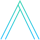# Dijkstra’s Algorithm in Kotlin

April 27, 2019

A while ago I wrote Dijkstra’s algorithm in Python, and I figured I’d convert it to Kotlin to test my Kotlin skills, since it’s a language I’m interacting with a lot at work these days.

Dijkstra’s algorithm answers the question – What is the shortest path from a start vertex to every other vertex in the graph? It hinges on the observation that any subpath of a shortest path must also be a shortest path (I encourage you to think about why). Here is my favorite explanation of it, and here is a useful visualization.

My code is more focused on education, mirroring the way I learned it originally at GT. To speed it up, you can make the operation of getting the next vertex to explore (v, in the code) done with a min heap.

Some other good implementations to compare with are Rosetta code, and the one in Kotlin Algorithm Club (what an awesome project!).

So, first let’s write a Graph class, so the algorithm has something to act upon.

.css-1baulvz{display:inline-block;}data class Graph<T>(    val vertices: Set<T>,    val edges: Map<T, Set<T>>,    val weights: Map<Pair<T, T>, Int>)

Without going too much into the details of initialization and providing a simple API, this is essentially my Graph class (on Github I add an additional constructor).

Now, let’s actually take a look at the algorithm.

fun <T> dijkstra(graph: Graph<T>, start: T): Map<T, T?> {    val S: MutableSet<T> = mutableSetOf() // a subset of vertices, for which we know the true distance    val delta = graph.vertices.map { it to Int.MAX_VALUE }.toMap().toMutableMap()    delta[start] = 0    val previous: MutableMap<T, T?> = graph.vertices.map { it to null }.toMap().toMutableMap()    while (S != graph.vertices) {        val v: T = delta            .filter { !S.contains(it.key) }            .minBy { it.value }!!            .key        graph.edges.getValue(v).minus(S).forEach { neighbor ->            val newPath = delta.getValue(v) + graph.weights.getValue(Pair(v, neighbor))            if (newPath < delta.getValue(neighbor)) {                delta[neighbor] = newPath                previous[neighbor] = v            }        }        S.add(v)    }    return previous.toMap()}fun <T> shortestPath(shortestPathTree: Map<T, T?>, start: T, end: T): List<T> {    fun pathTo(start: T, end: T): List<T> {        if (shortestPathTree[end] == null) return listOf(end)        return listOf(pathTo(start, shortestPathTree[end]!!), listOf(end)).flatten()    }    return pathTo(start, end)}

Definitely enjoy coding in Kotlin, it provides a lot of good API’s for functional programming.

Here’s the test:

@Testfun shouldCalculateCorrectShortestPaths() {    val weights = mapOf(        Pair("A", "B") to 2,        Pair("A", "C") to 8,        Pair("A", "D") to 5,        Pair("B", "C") to 1,        Pair("C", "E") to 3,        Pair("D", "E") to 2    )    val start = "A"    val shortestPathTree = dijkstra(Graph(weights), start)    Assert.assertEquals(listOf(start, "B", "C"), shortestPath(shortestPathTree, start, "C"))    Assert.assertEquals(listOf(start, "B", "C", "E"), shortestPath(shortestPathTree, start, "E"))    Assert.assertEquals(listOf(start, "D"), shortestPath(shortestPathTree, start, "D"))}

If you’d like to run the code, clone the git repo and get started using gradle.

# Join the mailing list

Get the latest posts in your inbox. No spam, ever.